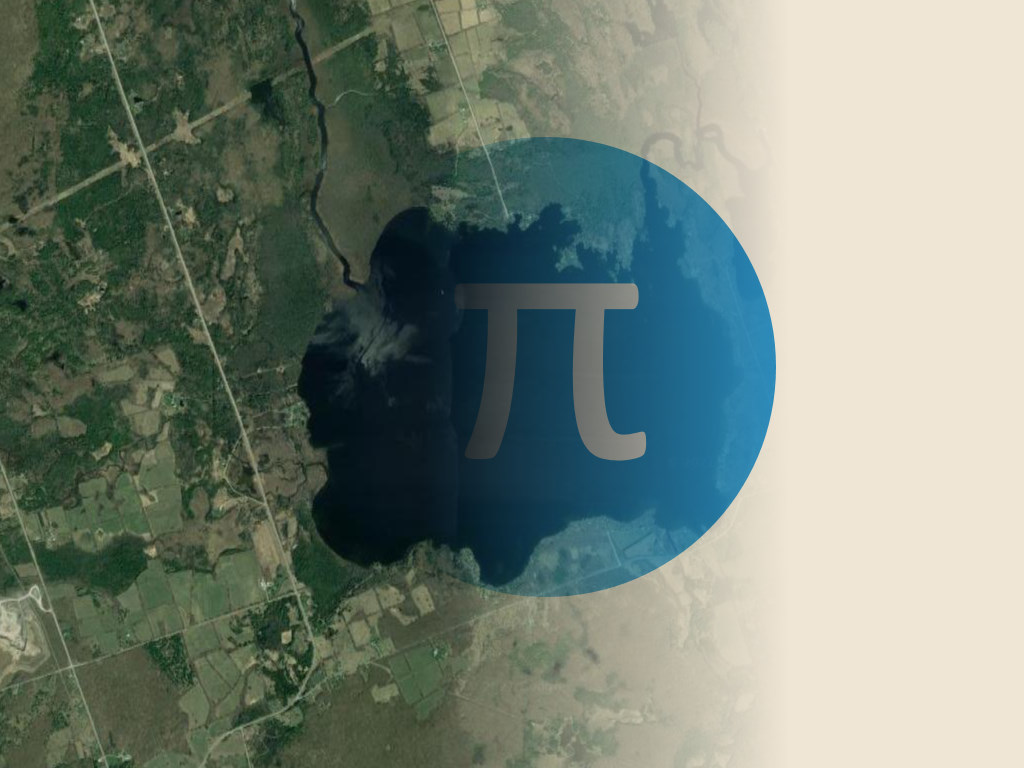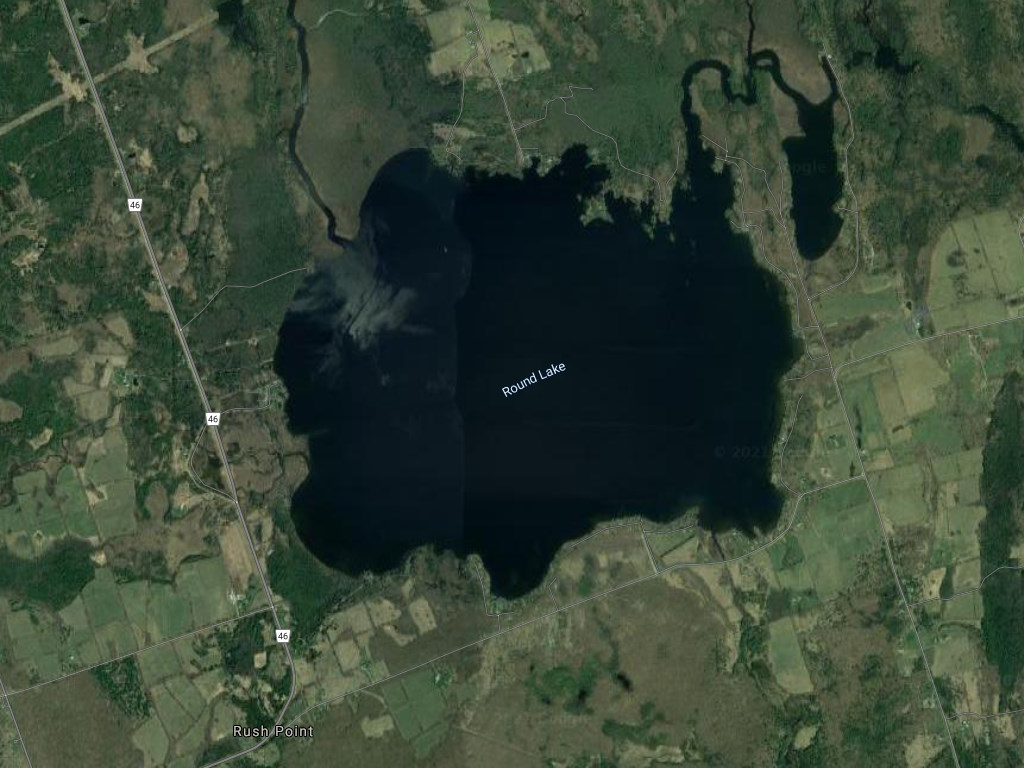# Calculating π Using Lakes from the Land BetweenHey folks! I know I’m late to the party, but hey I post on the last week of the month, so happy π day! In addition to environmental shenanigans, I also enjoy some good olde mathematics goofiness, especially when there is some great geometry involved. So in celebration of this year’s π day, I thought I’d try and find some lakes in the Land Between that will give us as close an approximation of π as possible working backwards from their surface area.

For those of you unaware, π day happens every year on March 14th (3/14) due to the numerical date’s similarity to the value of π (3.14….)

The first problem to overcome when calculating pi from a lake’s geometry is the shoreline fractal problem ie. the coastal paradox. Essentially, depending on the length of the ruler you use, you can get wildly different results. A shoreline is a curved fractal that looks very different depending on the scale at which you view it. From an airplaine, details on the shore you could see at ground level totally vanish, while details under a microscope reveal even more intricate patterns within the shoreline. All of this means that it is actually quite difficult to measure the actual length of a shoreline, take this exaggerated example:

Here is Little Lake in Peterborough. Using 2 different length rulers I get different results for the shoreline length. As I increase the detail, the length of the shoreline increases by 0.23km! Thankfully for us, the Ministry of Natural Resources produces standardized map layers we can use to calculate the area of different lakes. We’ll use the map layer meant to be viewed at 1:100,000 scale.

For those of you who need a refresher, the formula for calculating the area of a circle can be done with the following formula:$A =\pi r^2$

In order to calculate π we need to do some rearranging. For this problem the two givens we have are the lake’s “circumference” and it’s area. Unfortunately, this is where we need to fudge things a little bit. In order to calculate π we will need to have a radius in addition to the lake’s area. In order to calculate the radius we will take the average of 3 measurements of the lake’s diameter and divide by 2. So now are formula looks like this:$\pi = {A}\div{(\frac{r_{1}+r_{2} + r_{3}}{3}{)}^2}$

Now that we know what our formula is, lets go find some lakes to measure. For starters, lets try the obvious, Round Lake near Havelock. Its in the name so it must be pretty close to a circle!Is Round Lake the roundest? Stay tuned to find out!$\pi = {5,613,882m^2}\div{(\frac{1075m+1441m +1077m}{3}{)}^2}$

Plugging all of that into a calculator gives us a value of pi that is equal to 3.91! Pretty good for a natural object, but can we do better? Is Round Lake the roundest of the lakes?

I’ll spare you the math on this one. Longford Lake is in the middle of nowhere, I’ve never visited, but it sure looks nice from space! Maybe I’ll check it out someday if its pi enough.

With an average radius of 524m and an area of 1,140,948m2 North Longford clocks in at a pi value of 4.15. So sorry Longford, may you rest peacefully in continued obscurity.

Next up we’ll try Halls Lake north of Minden off Highway 35. Its a deep lake that is quite round with some nice trails nearby. Lets give it a shot!Halls Lake: Pretty round don’t ya think?$\pi = {5,354,281m^2}\div{(\frac{1453m+1360m +1181m}{3}{)}^2}$

All righty! We did the math (the monster math?) here at Stewards Notes and determined that Halls Lake is more round than Round Lake! Clocking in at a value of 3.02 for π! Congratulations Halls Lake! Be sure to visit Halls Lake some day and give a toast to how π it is.David Sumner says: